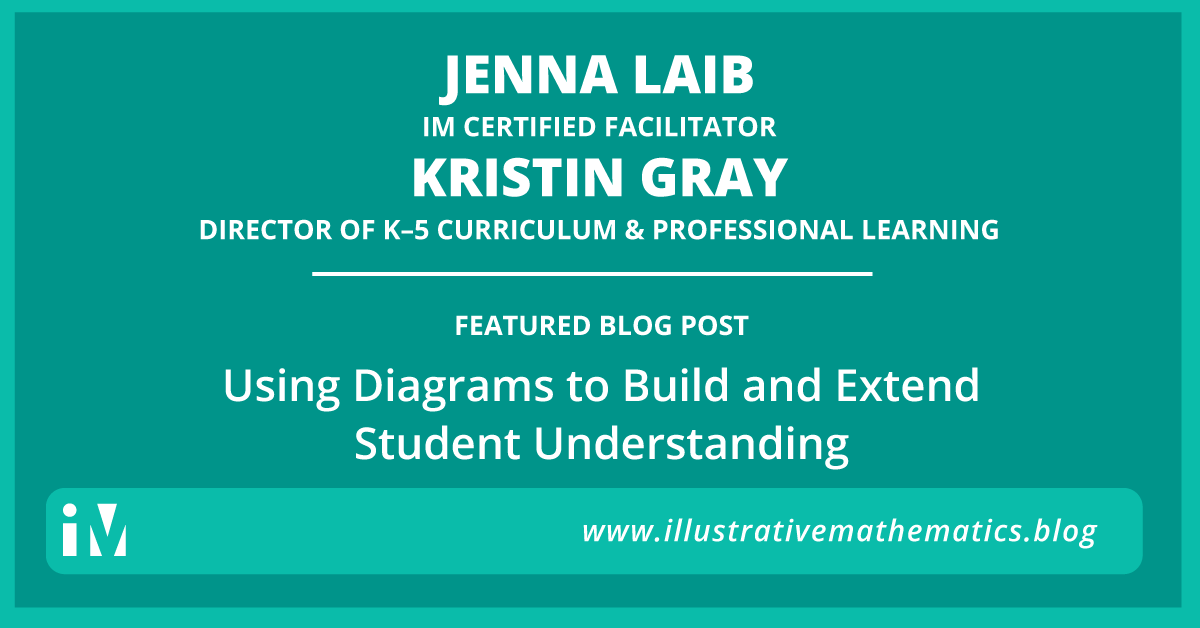## Using Diagrams to Build and Extend Student Understanding

By Jenna Laib and Kristin Gray Take a moment to think about the value of each expression below.  $\frac{1}{4}\times \frac{1}{3}$ $\frac{1}{4}\times \frac{2}{3}$ $\frac{2}{4}\times \frac{2}{3}$ $\frac{3}{4}\times \frac{2}{3}$ What do you notice? How would you explain...## Building a Math Community with IM K–5 Math

“I’m not sure this is working. Only five of my students are participating and commenting each day. The rest sit there and look at me.” By Tabitha Eutsler This was my conversation with our math coordinator after my first few days of teaching IM K–5 MathTM with my third...## Using Instructional Routines to Inspire Deep Thinking

We want students to think about math deeply. Creatively. Analytically. Instead, what often happens is that students race towards quick solutions. So what can we do to support this other kind of thinking in class—the slow, deep kind? By Jenna Laib One way is through...## Making Authentic Modeling Possible

The first thing you have to understand is that asking people to model with mathematics makes them mad. Not in all contexts, though! At a social gathering with a generally amiable and curious group of people, you might try floating a question like: I wonder if...## Concrete Representations that Give Students a Way to Get Started

This blog post is the third in a series of four blog posts exploring the student experience of problem-based learning. The first two posts are available here: “How Do Students Perceive Problem-Based Learning?” and “Inviting Students to the Mathematics.” By Max...